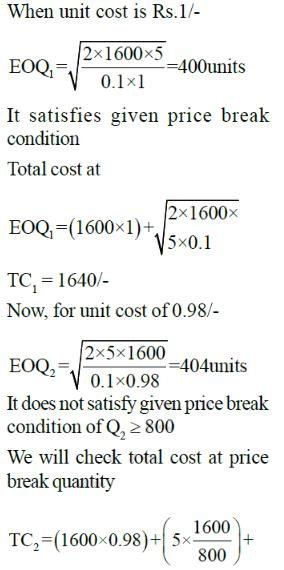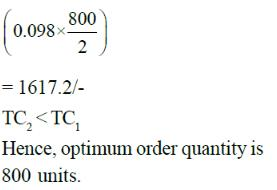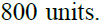Courses

# Industrial

## 25 Questions MCQ Test Mock Test Series - Mechanical Engineering (ME) for GATE 2020 | Industrial

Description
This mock test of Industrial for GATE helps you for every GATE entrance exam. This contains 25 Multiple Choice Questions for GATE Industrial (mcq) to study with solutions a complete question bank. The solved questions answers in this Industrial quiz give you a good mix of easy questions and tough questions. GATE students definitely take this Industrial exercise for a better result in the exam. You can find other Industrial extra questions, long questions & short questions for GATE on EduRev as well by searching above.
QUESTION: 1

### Consider the given LPP:-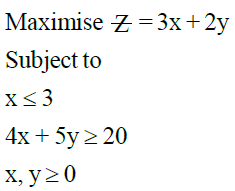Solution: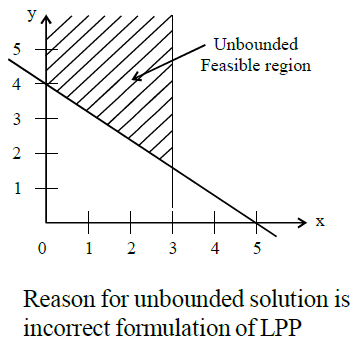QUESTION: 2

### In solution of transportation model of dimension m x n, degeneracy occurs when it has:-

Solution:

If basic feasible solution has less
than (m + n - 1) allocations, it leads
to degeneracy.

QUESTION: 3

### Four new machines M1, M2, M3 and M4 are to be installed in a machine shop. There are five vacant places A, B, C, D and E available. Because of limited space, M2 cannot be placed at C and M3 cannot be placed at A. Cost of placing of machine (in hundred rupees) is shown below:-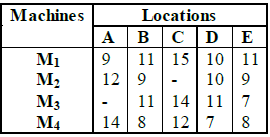Minimum total cost for optimal assignment schedule will be:-

Solution:

It is unbalanced assignment problem. Convert it into balanced problem by inserting dummy row and then proceed.

*Answer can only contain numeric values
QUESTION: 4

Common data for 4 & 5
Jobs are to be scheduled through one machine and details are given below:-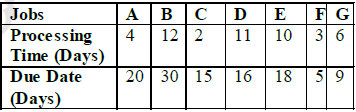Q. If first come, Fist Served (FCFS) rule is used to sequence the job, the number of jobs delayed is___

(Important - Enter only the numerical value in the answer)

Solution:

As per FCFS, sequence is
A-B-C-D-E-F-G
No. of jobs delayed are 5.

QUESTION: 5

Common data for 4 & 5
Jobs are to be scheduled through one machine and details are given below:-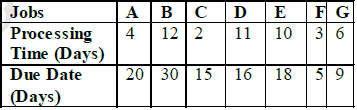Q. Using Shortest Processing Time (SPT) rule, total tardiness will be

Solution: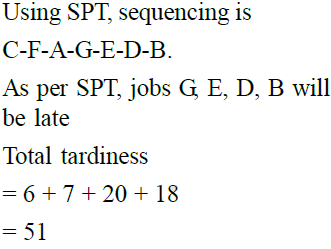QUESTION: 6

Breakdown occurs at average of 2 per hour in a machine in production shop. Cost of repairman is Rs.50/-
per hour and repair rate is 3 per hour. The average time that machine is waiting for the repairman will be:-

Solution: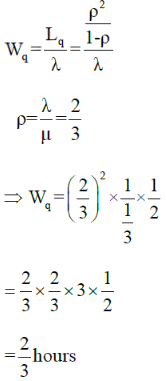QUESTION: 7

Consider the seven jobs, each of which has to go through the machines A and B in the order AB Processing time in hours are given in table below:-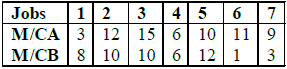Processing sequence that would minimise time span and the minimum elapsed time respectively are: -

Solution:

There are two optimal sequences
i.e.
1-4-5-3-2-7-6 or 1-5-3-2-4-7-6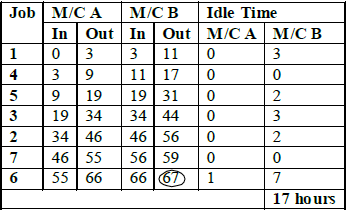QUESTION: 8

In (Q-7) Idle time for machine B is:-

Solution:

As per the table in previous  solution, idle time for M/C B is 17 hours.

*Answer can only contain numeric values
QUESTION: 9

Earliest completion time of the given project is _____days.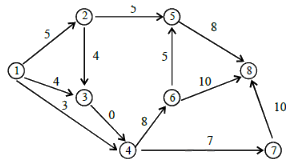(Important - Enter only the numerical value in the answer)

Solution:
QUESTION: 10

In LPP with two variable, if m is the number of constraints and nonnegativity constraints x > 0, y > 0,
the feasible region in the graphical solution will be surrounded by how many lines?

Solution:
QUESTION: 11

An investor is interested to invest in shares that has a growth of at least Rs.600/- initially with a dividend
of at least Rs.500/- per annum. He choose two shares S1 and S2. As per market study, share S1 will earn
dividend of 7% per annum and share S2 will earn 15% per annum.

Prediction is that growth in market value in first year share S1 will be 25 paise per rupee invested and in
case of share S2, 30 paise per rupee invested LPP is (x1 amount is invested in S1 and x2 in S2) :-

Solution: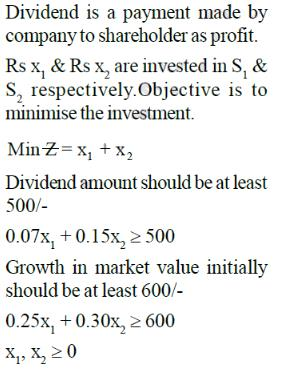QUESTION: 12

In a transportation problem, a solution is a basic feasible solution if after allocations

Solution:

Basic feasible solution will
contain (m + n - 1) independent
non-zero allocations. A feasible
solution to a transportation
problem is basic if the
corresponding cells in
transportation table do not
contain a closed loop.

QUESTION: 13

Four persons are to be assigned four tasks. Assignment problem is shown below. Optimum solution of problem is:-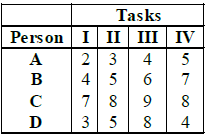(Table depicts the time taken by person to complete the task)

Solution:
QUESTION: 14

Project duration in CPM can be reduced by crashing:-

Solution:
QUESTION: 15

Consider the network shown below. Critical path is along :-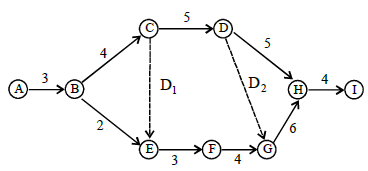Solution: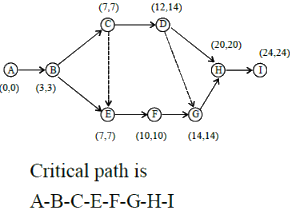*Answer can only contain numeric values
QUESTION: 16

At a self service store, cashier can serve 10 customers in 5 minutes as per exponential distribution. On an
average 15 customers arrive every 10 minutes as per Poission distribution. Probability that the cashier would be idle is_____.

(Important - Enter only the numerical value in the answer)

Solution: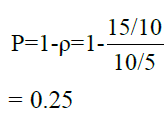QUESTION: 17

In a network, dummy activities are used to:-

Solution:
QUESTION: 18

In a network, the optimistic, most likely and pessimistic times (in weeks) are shown for each activity.
Variance of completion time for this network will be:-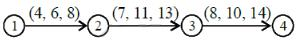Solution: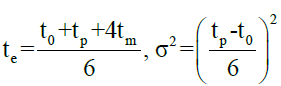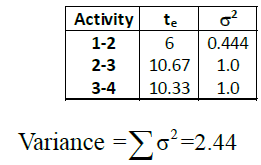QUESTION: 19

For the transportation problem shown below the optimum solution using VAM will be:-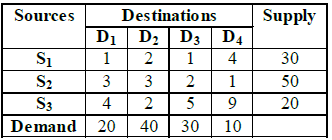Solution:
QUESTION: 20

Consider assignment problem as given below:-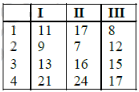For optimum assignment, associated minimum cost will be:-

Solution:
*Answer can only contain numeric values
QUESTION: 21

Company purchases an item at the rate of Rs2.5/- per piece from manufacturer. 25 units of item are required per year. Every time an order is placed, a fixed cost of Rs.4/ - is incurred.

Other charges are as: storage 1% per year, interest rate 12% per year and obsolescence rate 7% per year.

Total optimal variable cost is Rs___

(Important - Enter only the numerical value in the answer)

Solution: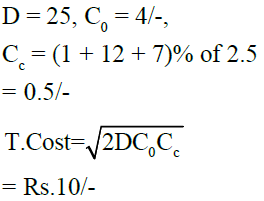QUESTION: 22

Firm requires 100 units per day of an item. It costs Rs.100/- to initiate order for purchasing. Item kept in storage is estimated to cost about Rs 0.02 per day. Lead time b/w placing and receiving order is 12 days.

What will be optimum inventory policy for ordering the part?

Solution: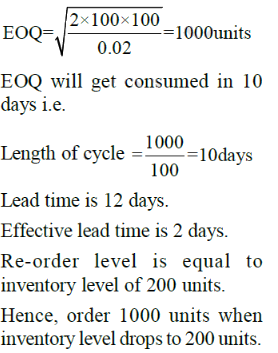QUESTION: 23

For a product, production rate is 50 items per day and demand rate is 25 items per day.

Set up cost is Rs.100/- per set-up and holding cost is Rs 0.01/- per unit per day. If shortages are not permitted, minimum total cost for one run will be:-

Solution: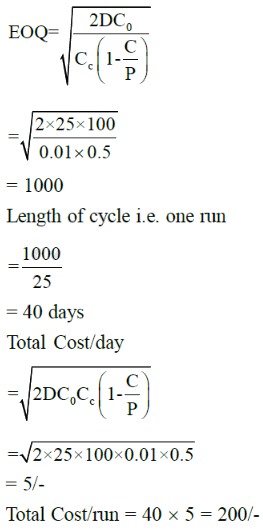QUESTION: 24

Sales of cycle in a shop in three consecutive months are given as 120, 100, 130. Exponential smoothening average method with  smoothening factor of 0.2 is used in forecasting. Expected no. of sales in next month is:-

Solution: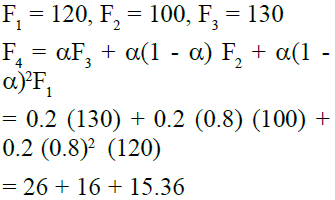= 57.36

QUESTION: 25

For a product, demand is 1600 units per year, cost of placing an order is Rs.5/- and cost of storage is 10% per year. Price breaks for the product are as follows:-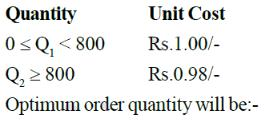Solution: